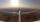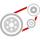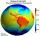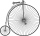# Perimeter + motion problems - examples

1. Rotation of the EarthCalculate the circumferential speed of the Earth's surface at a latitude of 61°​​. Consider a globe with a radius of 6378 km.
2. Earth's diameterThe Earth's diameter on the equator is approximately 12750 km. How long does the Gripen flyover the Earth above the equator at 10 km if it is at an average speed of 1500 km / h?
3. Engine pulleyThe engine has a 1460 rev / min (RPM). Disc diameter is 350 mm. What will be the disc peripheral speed in RPM? Pulleys on the engine has diameter 80mm, on a disc has diameter 160mm.
4. Average speedWhat is the average speed you have to move the way around the world in 80 days? (Path along the equator, round to km/h).
5. VelocipedesIn the 19th century, bicycles did not have a chain drive, and the pedals were connected directly to the wheel axis. This wheel diameter gradually increased until the so-called high bicycles (velocipedes) with a front wheel diameter of up to 1.5 meters, whi

We apologize, but in this category are not a lot of examples.
Do you have an interesting mathematical example that you can't solve it? Enter it, and we can try to solve it.

To this e-mail address, we will reply solution; solved examples are also published here. Please enter e-mail correctly and check whether you don't have a full mailbox.